# Logarithmic equations

Definition: Logarithmic equation — an equation in which the variable is under the sign of logarithm..

So good to be able rozwiazywanie logarithmic equations, you need to be able to control the reference ratio of the logarithm.

## Equivalent transformation of simple logarithmic equations.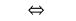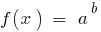Since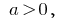theDHS and therefore the initial equation is automatically taken into account.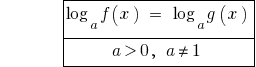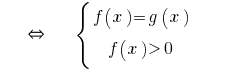or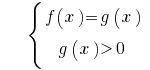## Examples of the solution of the simplest logarithmic equations

### Example 1

Rozwarte equation: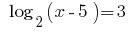Solutions: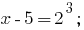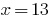Answer: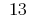### Example 2

Rozwarte equation: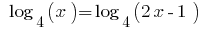Solutions:(DHS also considered)

Then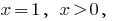that is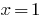Answer: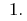## The scheme is more complex logarithmic equations

1. The use of equations and effect
2. Using the properties of the relevant functions
3. The use of equivalent transformations

## As razvesti logarithmic equation

Using the formulae of logarithm and potentiating reduce the equation to a simple (consider the initial DHS and make sure not to lose roots when sujuan DHS). After transformation, if it is not possible to reduce to a simple logarithmic equations we are trying to introduce a change of variables.

## Examples of the solution of logarithmic equations

### Example 3 (use of formulas, logarithms)

Rozwarte equation:Solutions:

Going to the base 2, we get the equivalent equation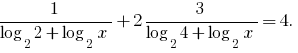Replacement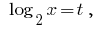Then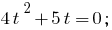Answer: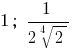### Example 4 (using properties of logarithmic functions)

Rozwarte equation: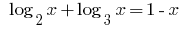Solutions:

The function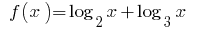increases in scopeas the sum of two increasing functions, and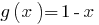comes. Therefore, the given equation has a single rootAnswer:Tags:
Chapter:
Versions in other languages:
Share with friends: